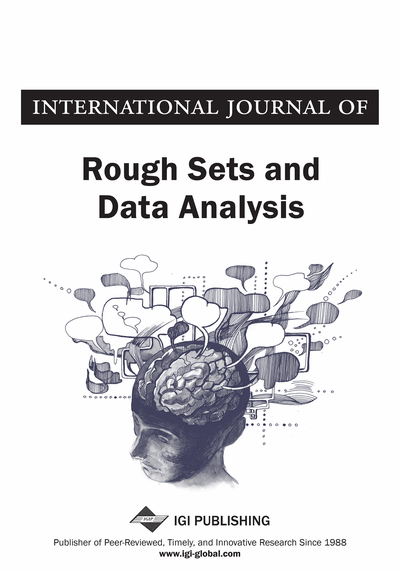# Comparing and Contrasting Rough Set with Logistic Regression for a Dataset

Renu Vashist (Shri Mata Vaishno Devi University, Katra, Jammu and Kashmir, India) and M. L. Garg (Shri Mata Vaishno Devi University, Katra, Jammu and Kashmir, India)
Copyright: © 2014 |Pages: 18
DOI: 10.4018/ijrsda.2014010106

## Abstract

Rough Set Theory (RST) is relatively new and powerful mathematical tool to deal with imperfect data (i.e. data with uncertainty and vagueness) which is primarily used for classification and decision making problems. On the other hand, Logistic regression (Logit) is mainly used in Social Sciences when dependent variable takes limited and categorical data value ranges. However, both RST and Logit regression are powerful predictable models that are used in wide range of applications such as medicine, military, banking, financial markets etc. RST uses approximations and implications as two formal tools to deal with vagueness whereas Logit regression is severely constrained to deal with vague and imprecise data. Yet, both these methodologies are used to classify the object which is the key issue in decision making. This research paper compares these two tools on a common dataset. SPSS 17.0 software is used to run the Logit regression and Rose 2 software is used for analysis of Rough Set. One of the important finding of this comparison is that attributes in core of the data set under the rough set approach are similar to the most significant predictors of logistic regression model. This indicates that the significant attributes deducted by these two methodologies are similar. It is demonstrated that rough set is much more superior tool to classify the objects as compared to logistic regression. One of the important outcomes of this research is that degree of accuracy is much higher in rough set as compared to logistic regression thereby establishing the supremacy of rough set as a better decision making tool.
Article Preview

## 1. Introduction

Logistic regression was proposed in the early 19th century for the description of the growth of population and it became frequently available in statistical packages in the early 1980s (Cramer, 2003). One of the basic assumptions of simple regression model is that the dependent variable is quantitative whereas the independent variable may be either quantitative or qualitative in nature (Haberman, 1978). In some other types of regression model the dependent variable can take only two values i.e. 1 or 0 or in other words, the dependent variable is ‘dichotomous’ in nature and ordinary least square (OLS) regression is incapable of handling such problems. Logistic regression is proposed as an alternative technique to overcome the limitation of OLS to handle dichotomous outcomes. There are many research problems in which the outcome can only assume two values like ‘yes’ or ‘no’ such as the patient is suffering from a disease or not. The logistic regression is suitable under those research problems where the independent variables are categorical, or a mix of continuous and categorical, and the dependent variable is categorical. This form of regression is often used when the relationship between independent and dependent variable is non linear. With the onset of sophisticated statistical software and high-speed computers, the applications of logistic regression have increased exponentially. The focal mathematical concept in logistic regression is the logit—the natural logarithm of an odds ratio. The only assumption is that the regression equation should have a linear relationship with the logit form of the dependent variable. There is no assumption about the predictors or independent variables being linearly related to each other. Logistic regression can accommodate categorical outcomes which are polytomous in nature however; this research paper focuses on dichotomous outcomes only. It is pertinent to mention that the logistic regression predicts the probability of an event’s outcome from a set of predictors (Demaris, 2013).

On the other hand rough set theory is based on the assumption that the every object of the universe is associated with some information i.e. data and information. Objects which are having the same information are indiscernible in view of the available information. The Indiscernibility relation is the mathematical basis of rough set theory (Pawlak, 1992).

RST basically operates on an information system which contains both quantitative and qualitative data. There are number of object in the information system and each objects has number of attributes which describe the object. RST has a unique ability to define uncertain objects in terms of certain definable objects using lower and upper approximation. Lower approximation contains objects that definitely belong to the set. The remaining objects are either definitely not in the set, or their set membership is unknown. The set of objects whose membership is unknown is called the boundary region. The upper approximation is the union of the lower approximation and the boundary region. Results of analyses using rough sets theory are usually presented as sets of rules linking attributes. Each rough set has boundary-line cases, i.e., objects which cannot be with certainty classified as members of the set or of its complement. Obviously crisp sets have no boundary-line elements at all. This means that boundary-line cases cannot be properly classified by employing the available knowledge. The difference between the upper and the lower approximation constitute the boundary region of the vague concept. Approximations are the two basic operations in rough set theory (Pawlak et al., 2007b).

## Complete Article List

Search this Journal:
Reset
Open Access Articles: Forthcoming
Volume 7: 4 Issues (2020): Forthcoming, Available for Pre-Order
Volume 6: 4 Issues (2019): 1 Released, 3 Forthcoming
Volume 5: 4 Issues (2018)
Volume 4: 4 Issues (2017)
Volume 3: 4 Issues (2016)
Volume 2: 2 Issues (2015)
Volume 1: 2 Issues (2014)
View Complete Journal Contents Listing